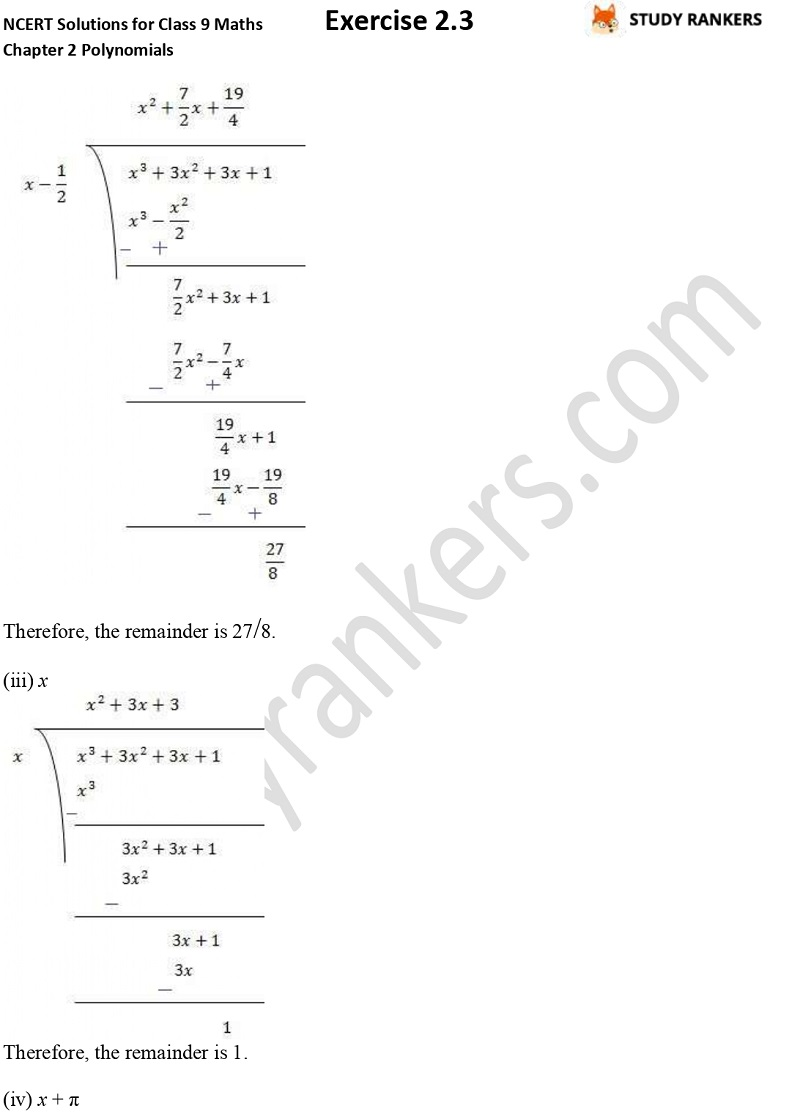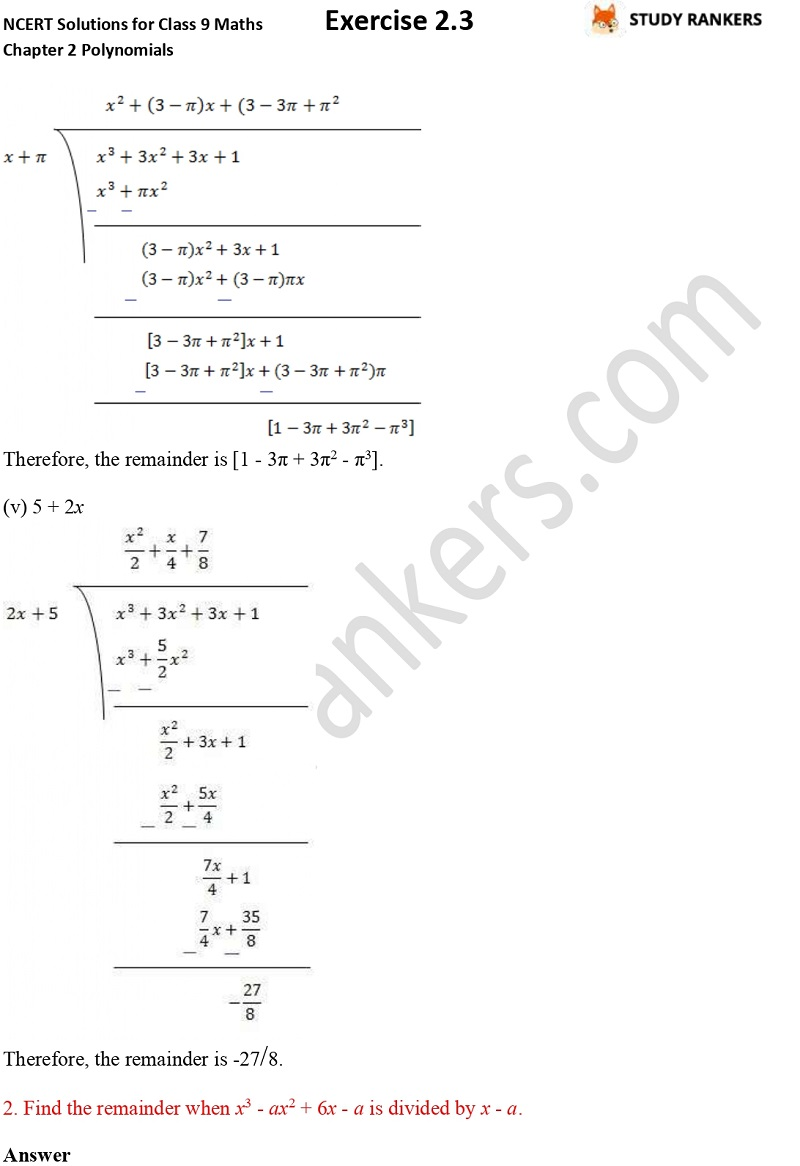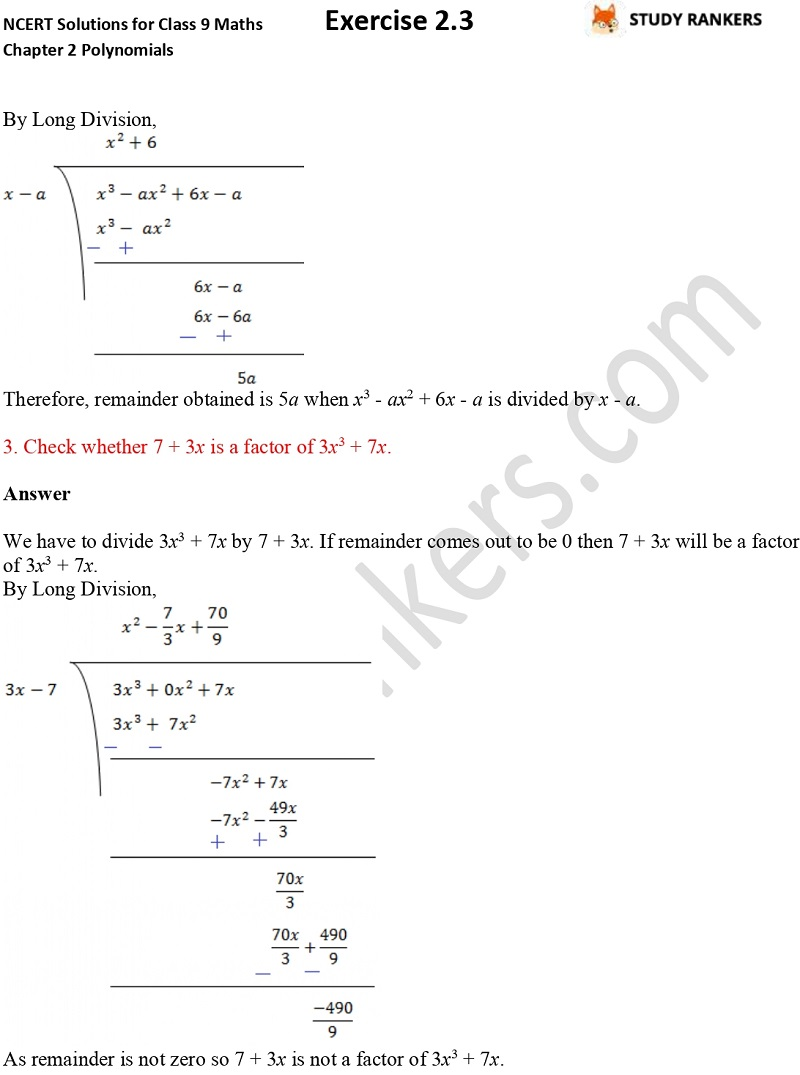## NCERT Solutions for Class 9 Maths Chapter 2 Polynomials Exercise 2.3

Chapter 2 Polynomials Exercise 2.3 Class 9 Maths NCERT Solutions is provided here will help you in understanding the fundamentals of the chapter. Class 9 Maths NCERT Solutions will be beneficial in completing homework and learn tips to solve maths questions in less time to score better marks in the examination. If you have face any types of problem trying to solve question then you can take help from this page.

Exercise 2.3 has total three questions which is about find the remainder and factor.